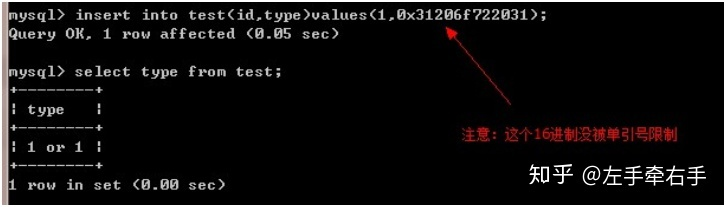# 写一个方法判断一个整数是否为质数_请写一个函数来检查用户提交的数据是否为整数...

1.is_integer 是 is_int() 的别名，是一样的

2.is_int/is_integer主要是判断变量是否 是整数类型，其他类型是false

3.is_numeric 检测变量是否为数字或数字字符串

$input ='12';//负整数 echo is_numeric($input ) ?'ok':'no'; //ok
echo is_int( $input ) ?'ok':'no'; //no  if(!is_numeric($input)||strpos($jp_total,".")!==false){ echo "不是整数"; }else{ echo "是整数"; }  is_numeric会引起sql注入问题 $s = is_numeric($_GET['s'])?$_GET['s']:0;
$sql="insert into test(type)values($s);";//是 values($s) 不是values('$s')
mysql_query(\$sql)04-03©️2020 CSDN 皮肤主题: 数字20 设计师:CSDN官方博客